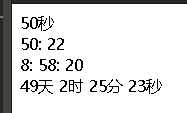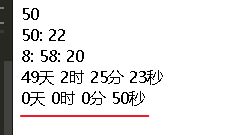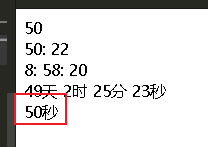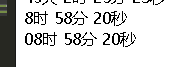• 时间换算毫秒换算成时间 直接调用就可以 transTime(value) { let time = ""; value /= 1000; let m = parseInt(value / 60); m = m < 10 ? "0" + m : m; let s = parseInt(value % 60); s = s < 10 ?...
时间换算 将毫秒换算成时间
直接调用就可以
transTime(value) {
let time = "";
value /= 1000;
let m = parseInt(value / 60);
m = m < 10 ? "0" + m : m;
let s = parseInt(value % 60);
s = s < 10 ? "0" + s : s;
time = m + ":" + s;
return time;
},

展开全文• 时间换算:        1s【秒】 = 1000ms【毫秒】   1ms【毫秒】 = 1000μs【微秒】    1μs【微秒】 = 1000ns【纳秒】   1ns 【纳秒】= 1000ps【皮秒】 1秒(s) = 1000 毫秒(ms) = ...
时间单位：
秒（second），时间单位 ： s，   毫秒(millisecond），时间单位：ms   微秒（microsecond），时间单位:μs
时间换算:
1s【秒】 = 1000ms【毫秒】   1ms【毫秒】 = 1000μs【微秒】    1μs【微秒】 = 1000ns【纳秒】   1ns 【纳秒】= 1000ps【皮秒】
1秒(s) = 1000 毫秒(ms) = 1,000,000 微秒(μs) = 1,000,000,000 纳秒(ns) = 1,000,000,000,000 皮秒(ps)

1秒 = 1000 毫秒 = 1000000 微秒 = 1000000000 毫微秒 = 1000000000 纳秒 = 1000000000000 皮秒 又可表示为： 1s = 1000 ms = 1000000 us = 1000000000 ns = 1000000000000 ps

扩展知识：
中国传统的计数单位有：

个十百千万亿兆京垓 分厘毫丝忽微

至万以后，亿有两种不同的意义，即10万或万万；（现已不用前一意义）  兆也有两种不同的意义，即百万或亿万；（现两种意义都在使用。）  类似地，京为千万或兆万；垓为万万或京万。（现已不使用京和垓的单位）。  即至万以后，有用十进位，但通常是万进位。
在法定计量单位中，用词头计数：

十、百、千、兆、吉、太、拍、艾、泽、尧。

千以前是十进位，千以后是千进位。

分、厘、毫、微、纳、皮、飞、阿、仄、夭。

展开全文linux
• 毫秒转换成日常使用的时间格式
一、53200毫秒
只到秒，没有分，没有进位，只要简单的除以1000就可以了
{{Math.round(time0/1000)}}
二、3022222毫秒
进位到分钟，需要除以60,然后把小数点前后部分的数值分开来处理
				{{Math.floor(Math.round(time/1000)/60)}}:
{{Math.round(time/1000)%60}}
三、32300432毫秒
进位到了小时，需要把除以60得到的整数部分，再除以60，把得到的数的小数和整数部分分开继续处理
			{{Math.floor(Math.floor(Math.round(time2/1000)/60)/24) }}:
{{Math.floor(Math.round(time2/1000)/60)%24 }}:
{{Math.round(time2/1000)%60}}
四、4242323234毫秒
分步除以60、60、24，一共要处理四个数
			{{Math.floor(Math.floor(Math.floor(Math.round(time3/1000)/60)/60)/24)}}天
{{Math.floor(Math.floor(Math.round(time3/1000)/60)/60)%24}}时
{{Math.floor(Math.round(time3/1000)/60)%60}}分
{{Math.round(time3/1000)%60}}秒
显示结果：五、53200毫秒
把第一步的数和第四步的代码结合起来看看，出来的是什么结果这样显示明显不合适，0的时候就可以不用显示
加个v-show来做下判断，代码就改进成下面这样
给div都加上display: inline-block;改成行内块，让它们能显示在同一行
            <div>
<div v-show="time0/1000>86400" style="display: inline-block;">
{{Math.floor(Math.floor(Math.floor(Math.round(time0/1000)/60)/60)/24)}}天
</div>
<div v-show="time0/1000>3600" style="display: inline-block;">
{{Math.floor(Math.floor(Math.round(time0/1000)/60)/60)%24}}时
</div>
<div v-show="time0/1000>60" style="display: inline-block;">
{{Math.floor(Math.round(time0/1000)/60)%60}}分
</div>
{{Math.round(time0/1000)%60}}秒
</div>
显示就正常了再加个三目运算把个位数的前面都拼接上0
然后代码一下就变得无比的长
			<div>
<div v-show="time0/1000>86400" style="display: inline-block;">
{{Math.floor(Math.floor(Math.floor(Math.round(time0/1000)/60)/60)/24)<10?('0'+Math.floor(Math.floor(Math.floor(Math.round(time0/1000)/60)/60)/24)):Math.floor(Math.floor(Math.floor(Math.round(time0/1000)/60)/60)/24)}}天
</div>
<div v-show="time0/1000>3600" style="display: inline-block;">
{{Math.floor(Math.floor(Math.round(time0/1000)/60)/60)%24<10?('0'+Math.floor(Math.floor(Math.round(time0/1000)/60)/60)%24):Math.floor(Math.floor(Math.round(time0/1000)/60)/60)%24}}时
</div>
<div v-show="time0/1000>60" style="display: inline-block;">
{{Math.floor(Math.round(time0/1000)/60)%60<10?('0'+Math.floor(Math.round(time0/1000)/60)%60):Math.floor(Math.round(time0/1000)/60)%60}}分
</div>
{{Math.round(time0/1000)%60<10?('0'+Math.round(time0/1000)%60):Math.round(time0/1000)%60}}秒
</div>
显示效果对比重点：
%：取余
Math.floor()：相当于省去小数后的数字
Math.round()：四舍五入 
展开全文vue.js javascript
• 1s=1000ms=1000000微秒=1000000000纳秒=1000000000000皮秒=10^15飞秒=10^18啊秒=10^21仄秒=10^...1s=10^3ms(毫秒)=10^6μs(微秒)=10^9ns(纳秒)=10^12ps(皮秒)=10^15fs(飞秒)=10^18as(阿秒)=10^21zm(仄秒)=10^24ym(幺秒)

1s=1000ms=1000000微秒=1000000000纳秒=1000000000000皮秒=10^15飞秒=10^18啊秒=10^21仄秒=10^24幺秒

1s=10^3ms(毫秒)=10^6μs(微秒)=10^9ns(纳秒)=10^12ps(皮秒)=10^15fs(飞秒)=10^18as(阿秒)=10^21zm(仄秒)=10^24ym(幺秒)


展开全文• 1秒=1000毫秒(ms), 1毫秒=1／1000秒(s)；1秒=1000000 微秒(μs), 1微秒=1／1000000秒(s)；1秒=1000000000 纳秒(ns),1纳秒=1／1000000000秒(s)；1秒=1000000000000皮秒 1皮秒==1/1000000000000秒。   转自： ...
• 秒也是一个的时间单位，0.000 000 001 毫秒 = 1皮秒，0.000 001 毫秒 = 1纳秒，0.001 毫秒 = 1微秒，1毫秒等于0。001秒，60秒等于1分钟，60分钟等于1小时，24小时等于1天，7天等于1星期，30，31，28或29天等于1月，...
• 秒，时间单位，符号s(英语：second)：0.000 000 001 毫秒 = 1皮秒0.000 001 毫秒 = 1纳秒0.001 毫秒 = 1微秒1毫秒=0.001秒60秒=1分钟60分钟=1小时24小时=1天7天=1星期30，31，28或29天=1月12月=1年100年=1世纪。...
• Chrome控制台时间转换超级方便： 步骤1：打开Chrome浏览器，点击右键“审查元素” 步骤2：切换到“Console”控制台标签 步骤3：输入如下代码： 【1】生成当前时间戳： 代码：new Date().getTime() ...
• 1s(秒)=10^3ms(毫秒)=10^6μs(微秒)=10^9ns(纳秒)=10^12ps(皮秒)=10^15fs(飞秒)=10^18as(阿秒)=10^21zm(仄秒)=10^24ym(幺秒)  秒 seconds  毫秒 millisecond 微秒 microseconds 纳秒 nanosecond...
• 毫秒 换算成(天 时 分 秒 毫秒) 啥也不多说了，直接贴代码，需要的朋友可以拿去直接用，代码比较简单JAVA string
• 纳秒微秒毫秒 秒 ns、us、ms、s 时间单位之间的换算 1000000000纳秒=1秒 （9个0） 1000000微秒=1秒 （6个0） 1000毫秒=1秒 （3个0）数学
• 字符串类型日期换算毫秒数： String dateStr = "20201105182535"; SimpleDateFormat simpleDateFormat = new SimpleDateFormat("yyyyMMddHHmmss");//24小时制 long callTime = simpleDateFormat.parse...
• 秒，时间单位，符号s(英语：second)： 0.000 000 001 毫秒 = 1皮秒 0.000 001 毫秒 = 1纳秒 0.001 毫秒 = 1微秒 1毫秒=0.001秒 60秒=1分钟 60分钟=1小时 24小时=1天 7天=1星期 30，31，28或29天=1月 12...
• 1秒(s) 100分秒(ds)= 1000 毫秒(ms) = 1,000,000 微秒(μs) = 1,000,000,000 纳秒(ns) = 1,000,000,000,000 皮秒(ps) =1,000,000,000,000,000飞秒（fs)=1,000,000,000,000,000,000仄秒（zm)=1,000,000,000,
• 时间单位换算表与计算机单位换算时间单位换算表 与 计算机单位换算时间单位换算表1秒=1000毫秒(ms)1毫秒=1／1,000秒(s)1秒=1,000,000 微秒(μs)1微秒=1／1,000,000秒(s)1秒=1,000,000,000 纳秒(ns)1纳秒=1／1,000,...
• ## 时间单位换算

千次阅读 2015-08-28 13:13:47
时间单位还有：毫秒(ms)、微秒(μs)、纳秒(ns)、皮秒(ps)、飞秒(fs)、阿秒、渺秒1 s = 10^3 ms = 10^6 us = 10^9 ns = 10^12 ps = 10^15 fs=10^18阿秒=10^21渺秒=10^43普朗克常数毫秒毫秒是一种较为微小的时间单位，...
• ## 时间的单位换算

千次阅读 2013-08-26 08:23:16
时间的单位换算 秒 > 毫秒 > 微秒 > 纳秒 > 皮秒 s > ms > us > ns > ps 1 > 10^3 > 10^6 > 10^9 > 10^12 秒：second  毫秒：millisecond （milli 是毫，...
• 时间的单位换算： 1小时=60分， 1分=60秒， 1秒=1000毫秒
• 换算时间单位换算表 与 计算机单位换算时间单位换算表1秒=1000毫秒(ms)1毫秒=1／1,000秒(s)1秒=1,000,000 微秒(μs)1微秒=1／1,000,000秒(s)1秒=1,000,000,000 纳秒(ns)1纳秒=1／1,000,000,000秒(s)1秒=1,000,000,......